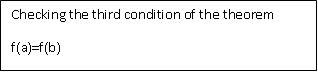## Rolle's theorem in Python

Check how to implement Rolle's theorem in Python:

In calculus, Rolle's theorem or Rolle's lemma basically means that any differentiable function of the realizable value that reaches the same value at two different points must have at least one stationary point somewhere between the two, that is, a point The derivation (slope) of the tangent to the graph of the function is equal to zero.

The extreme value theorem states that a continuous function must have a minimum and a maximum in a closed interval. These extremes may occur within or at the end of the closed interval. Rolle's theorem states that under certain conditions, an extreme value is guaranteed within the closed interval.

If an actual function f is continuous in a correct closed interval [a, b], distinguishable after the open interval (a, b) and f (a) = f (b), there is at least one c in the open interval (a, b) as

This version of the set of roles is used to demonstrate the set of average values, the set of roles that constitute a special case. It is also the basis of the proof of Taylor's theorem.

## ROLLE'S THEOREM - Conditions:

1. The function f must be continuous in the closed interval [a, b].

2. The function f must be differentiable in the opening interval (a, b).

3. f (a) = f (b)

If all three conditions are met then there is at least one number in the open interval (a, b) such that the derivative of f is zero.

In this section, we discuss how Rolle's Theorem work in Python. We implement the equation of Rolle's theorem using python code and satisfied all three conditions on the given interval.

1.IIIustrate the geometric meaning of Rolle’s theorem of the following function on the given interval:

f(x) = x3- 4x at [-2,2]
`Python Code:`
```from sympy import *
from random import randrange
import cmath
x,y,z = symbols('x y z')
init_printing(use_unicode=True)
flagcontinuity = False```
`flagdifferentiability = False`
`flagequality =  False`
```def h(x):
expr = x**3-4*x
return expr
print(h(x))
plot(h(x),(x,-2,2))```
`Output:`
`x3- 4x````
print('checking the first condition of the theorem')
lnhdlimit_pos = limit(h(x),x,-2,'+')
lnhdlimit_neg = limit(h(x),x,-2,'-')
print(lnhdlimit_pos)
print(lnhdlimit_neg)
rnhdlimit_pos = limit(h(x),x,2,'+')
rnhdlimit_neg = limit(h(x),x,2,'-')
print(rnhdlimit_pos)
print(rnhdlimit_neg)
if(lnhdlimit_pos==lnhdlimit_neg)and(lnhdlimit_neg==h(-2))and ```
```(rnhdlimit_pos==rnhdlimit_neg)and(rnhdlimit_neg==h(2)):
print('function is continuos')
else:
print("function is discontinuos")
flagcontinuity = True```
```Output:print('checking the second condition of theorem')
```
```for i in range(0,1):
j = randrange(-2,2)
difflnhd =diff(limit(h(x),x,j,'+'))
diffrnhd = diff(limit(h(x),x,j,'-'))
print(difflnhd)
print(diffrnhd)
if(difflnhd==diffrnhd):
print('function is differentiable')
else:
print('function is not differentiable')
flagdifferentiability = True```
`Output:``print('Checking the third condition od the theorem')`
```r1 = h(-2)
r2 = h(2)
if(r1==r2):
print('f(a)=f(b)')
else:
print('f(a)!=f(b)')
flagequality = True```
```Output:```
```if(flagcontinuity==True)or(flagdifferentiability==True)or(flagequality==True):
print("all conditions of rolles theorem is not satisfied")
else:
print("all conditions of rolles theorem is satisfied")
print("find the derivative of the function")
d1 = diff(h(x),x)
print('derivative of h(x) is',d1)
print('so there exist some constant c')
print('enter the value of a,b,c')
a = int(input())
b = int(input())
c = int(input())
disc = b**2-4*a*c
root1 = -b-cmath.sqrt(disc)/(2*a)
root2 = -b-cmath.sqrt(disc)/(2*a)
print('the derivative of equation are{0} and {1}'.format(root1,root2),'where derivative is zero' )
plot(h(x),(x,-2,2),root1,root2)
Output:``````
```
```
```Summary: In this section, we discuss the Rolle's theorem, conditions of the Rolle's theorem. In this first, we import all libraries that are necessary for the implementation of Rolle's theorem. You may also check the Python Libraries tutorial if you want to know how to import these libraries. Sympy stands for Symbolic Python so in this case we from sympy import *. This means it includes all the modules all in Sympy. then we impost cmath library which is necessary when we implement mathematical equations. We used lnhdlimit_pos (left neighborhood limit) and rnhdlimit_pos (right neighborhood limit) for both positive and negative. Then we check all the conditions of Rolle's theorem one by one using Python Code. If all three conditions satisfied then find the derivative of the function at a value equal to zero and plot the graph of the given equation.